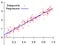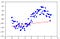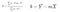Prerequisite : Different types of machine learning.

Linear Regression: it is a linear model that establishes the relationship between a dependent variable y(Target) and one or more independent variables denoted X(Inputs).Regression fits the data

## Goal is to find that blue straight line (which is best fit) to the data.

Our Training Data consists of X and y values so we can plot them on the graph, that’s damn easy. now what’s next? how to find that blue line????

First lets talk about how to draw a linear line in the graph,

In math we have an equation which is called linear equation

`y = mX+b { m->slope , b->Y-intercept }`

so we can draw the line if we take any values for m and b

How do we get the m and b values ??? and how do we know exact m and b values for the best fit line??

Lets take a simple data set (sine wave form -3 to 3) and First time we take random values of m and b values and we draw a line something like this.Random line for m and b

How we drew the above line?

we take the first X value(x1) from our data set and calculate y value(y1)

`y1=m*x1+b {m,b->random values lets say 0.5,1           x1->lets say -3 (first value from our data-set)y1=(0.5 * -3) + 1y1=-0.5 by applying all x values for m and b values we get our first line.Above picture has its own random variables ( I hope you understand the concept)`

That line is not fitting well to the data so we need to change m and b values to get the best fit line.

How do we change m and b values for the best fit line??

Either we can use an awesome algorithm called Gradient Descent (Which I will cover in next story with also the math used in there.)

Update:Here is the Gradient Descent Story

Or we can borrow direct formulas from statistics(they call this Least Square Method) I will also cover if possible in next story.X^ is mean of X values , Y^ mean of y values

Right now lets black box, we assume that we are getting the m and b values, Every time when the m and b values change we may get a different line and finally we get the best fit line

Pretty cool right?

So What’s next??? Predicting new data, remember?? so we give new X values we get the predicted y values how does it work ??

same as above y= m X +b , we now know the final m and b values.

This is called simple linear regression as we have only one independent X value. Lets say we wanna predict housing price based the size of house

X= Size (in sqft’s) y= Price (in dollar’s)

`X        y1000      402000      70500       25............`

What if we have more independent values of X????

Lets say we wanna predict housing price not only by the size of house but also by no of bedrooms

x1= Size (in sqft’s), x2=N_rooms and y= Price (in dollar’s)

`x1   x2   y1000  2    502000  4    90500   1    35............`

The process same as above but the equation changes a bit

Note: Lets alias b and m as θ0 and θ1 (theta 0 and theta 1 ) respectively.

y = θ0+θ1*X → b+mX → Simple LR → Single variable LR

y=θ0+θ1*x1+θ2*x2+..θn*xn → Multiple LR → Multi variable LR

Now we can predict as many things as we wish.

That’s it for this story , Hoping it helps at least 1 person.

In the next story I will talk about Gradient Descent Algorithm.

until then See ya!

Written by

## Deep Math Machine learning.ai

#### This is all about machine learning and deep learning (Topics cover Math,Theory and Programming)

Welcome to a place where words matter. On Medium, smart voices and original ideas take center stage - with no ads in sight. Watch
Follow all the topics you care about, and we’ll deliver the best stories for you to your homepage and inbox. Explore
Get unlimited access to the best stories on Medium — and support writers while you’re at it. Just \$5/month. Upgrade